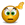IF Formula to calculate actual time*cost ?

Excel Help for If Formula To Calculate Actual Time*cost ? in Developing Business Administration Solutions

If Formula To Calculate Actual Time*cost ?

Rate this:
(3/5 from 1 vote)Hi,

I need some help working out how i can get my spreadsheet to understand that working for 15 minutes would be one price working for 30 mins would be double that price, this is for a company work log so the user would select i.e 1 hour in time taken and the total would calculate the cost.

ThanksRate this: (3/5 from 1 vote) If the time entered is in date/time format you get the number of hours by using the =HOUR(value) function. If you have a 15 minute price then you can simply divide the hours by 4 and multiply by the price. If you have multiple prices depending on time taken, then you can pass the time taken in a lookup function to get the rate and then multiply. Excel Business Forums Administrator Posted by Excel Helper on 16 Jun 2011